Not a member yet?Diagrams, Forms, & Illustrations

Models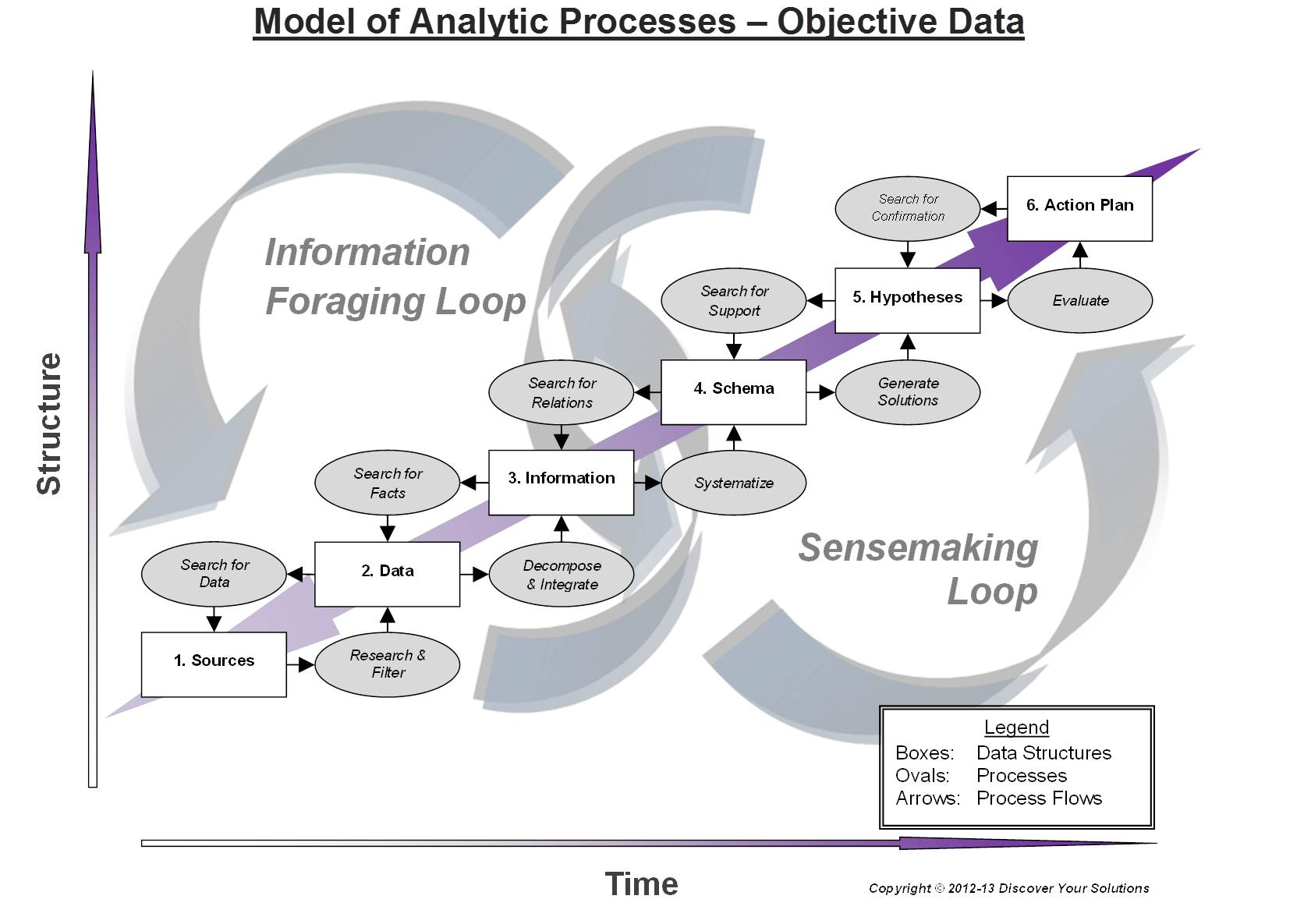Click to go to our Model of Analytic Processes - Objective Data (illustration)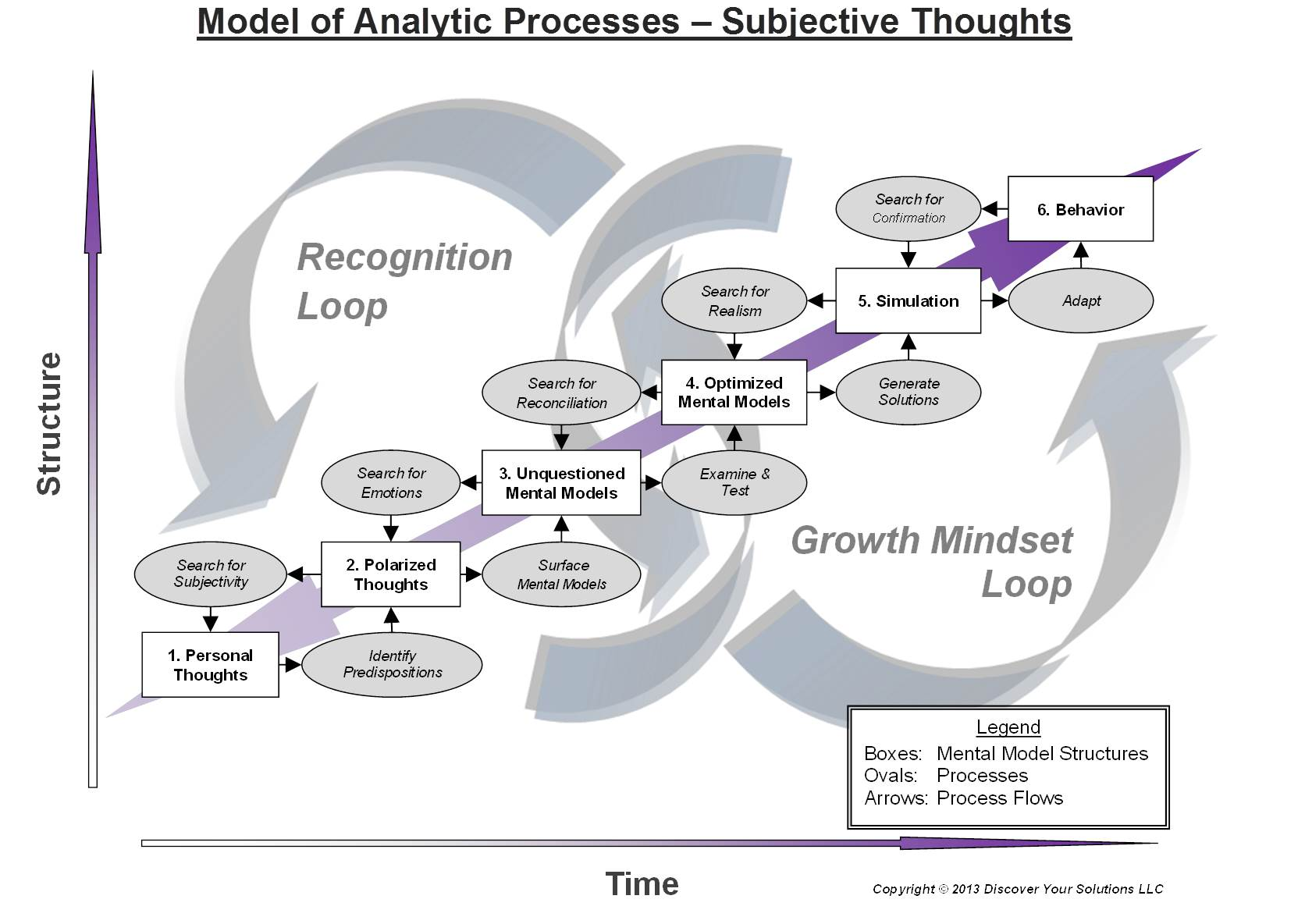Click to go to our Model of Analytic Processes - Subjective Thoughts (illustration)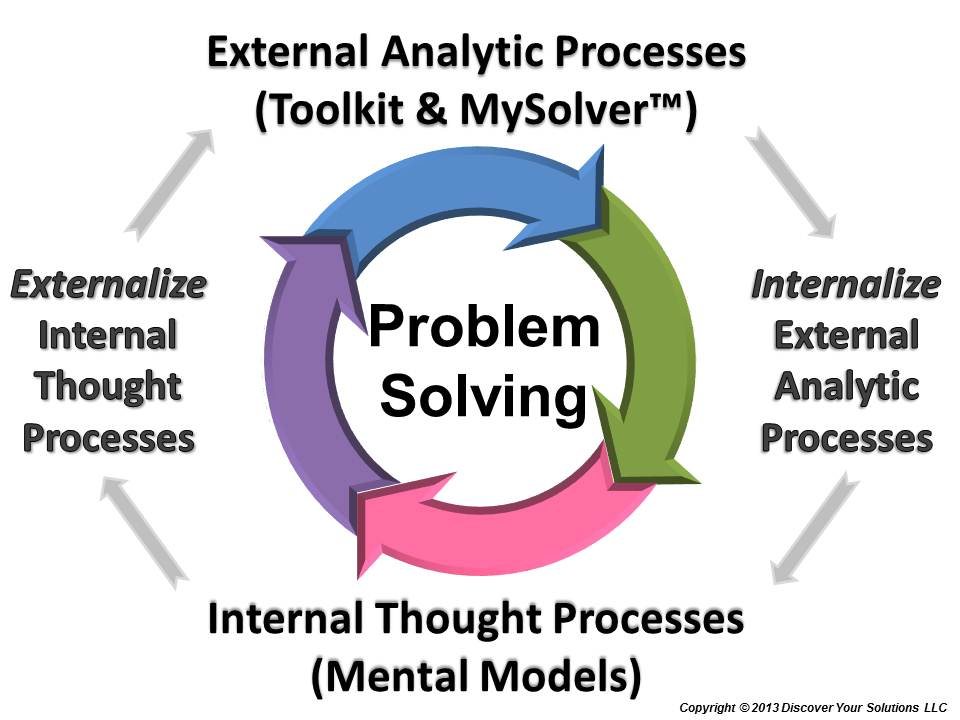Click to go to our Problem Solving Model (illustration)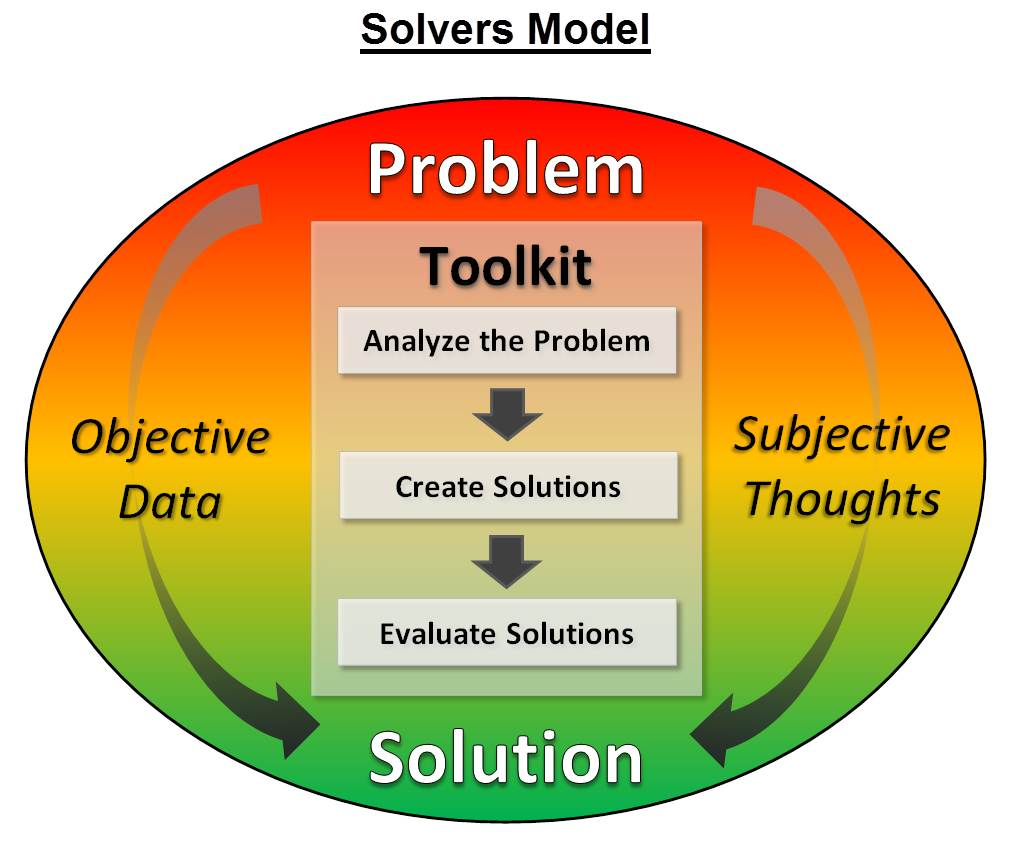Click to go to our Solvers Model (illustration)

Tools, Techniques, & Methods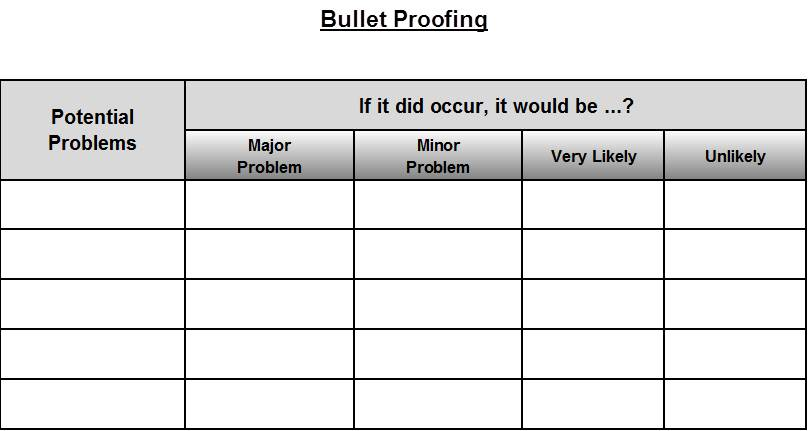Click to go to our Bullet Proofing (diagram)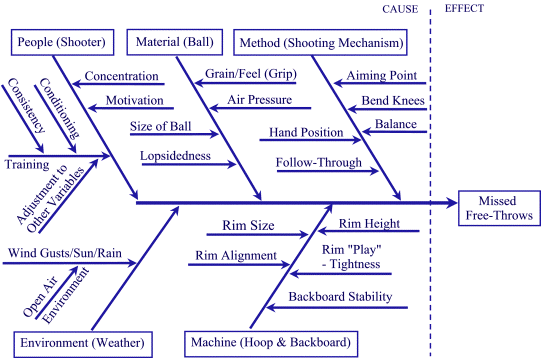Click to go to our Cause and Effect - Fishbone Diagram (diagram)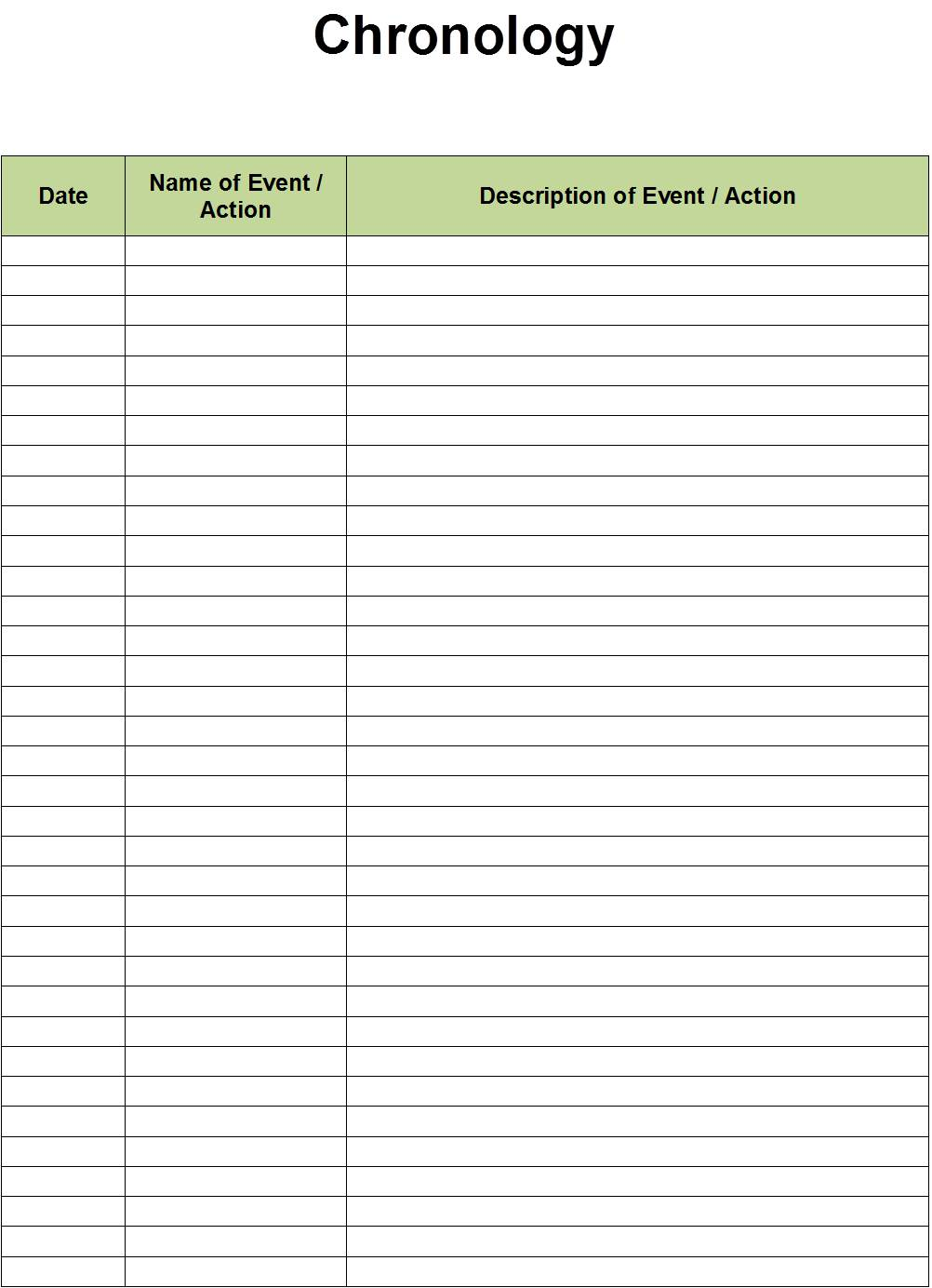Click to go to our Chronology (diagram)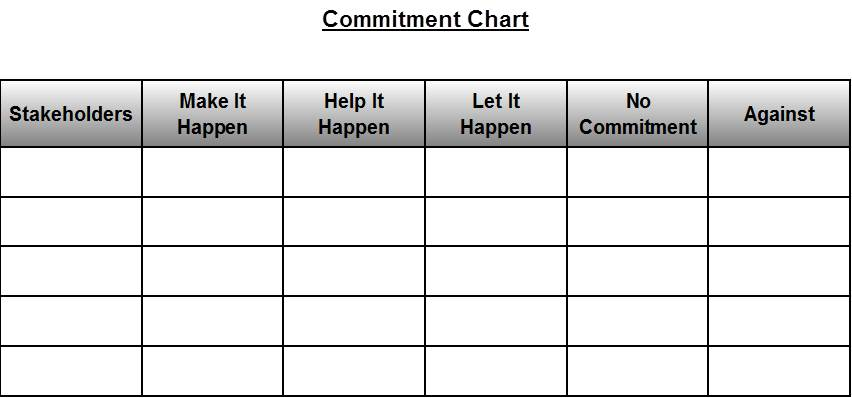Click to go to our Commitment Chart (diagram)Click to go to our Cross Impact Matrix (diagram)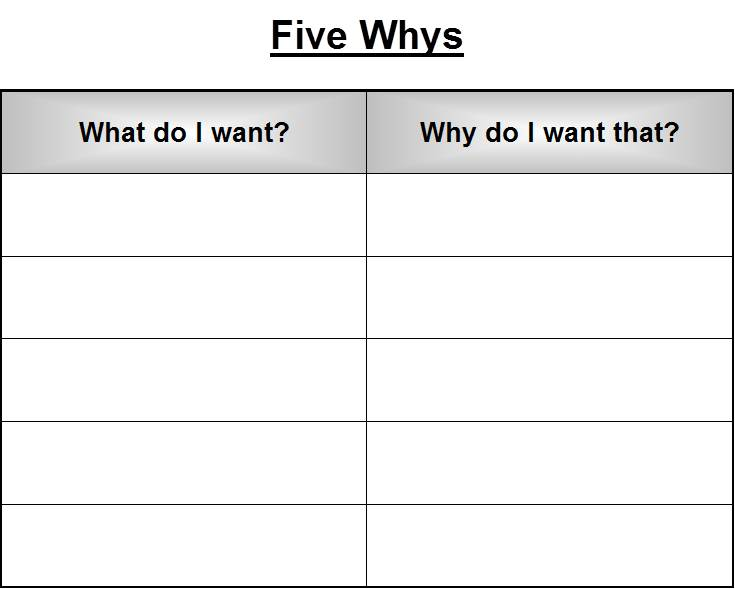Click to go to our Five Whys (diagram)Click to go to our Five Whys Alternative (diagram)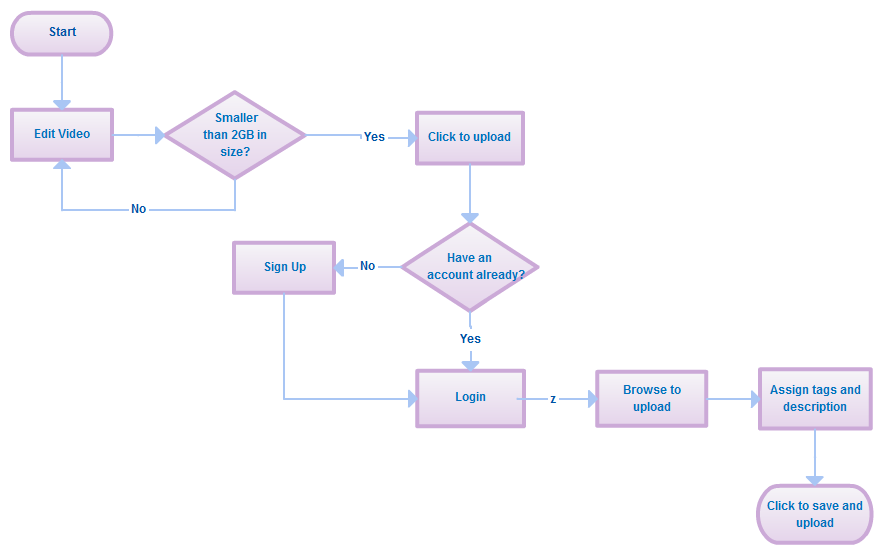Click to go to our Flow Chart (diagram)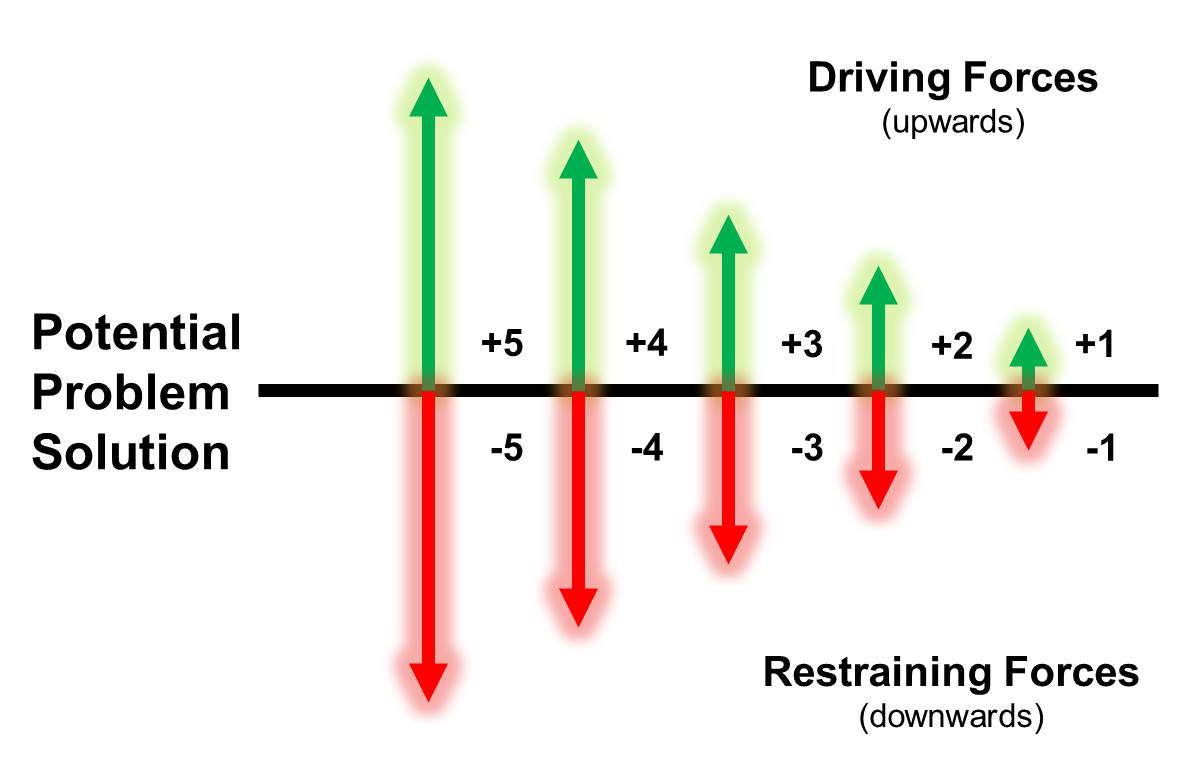Click to go to our Force Field Analysis (diagram)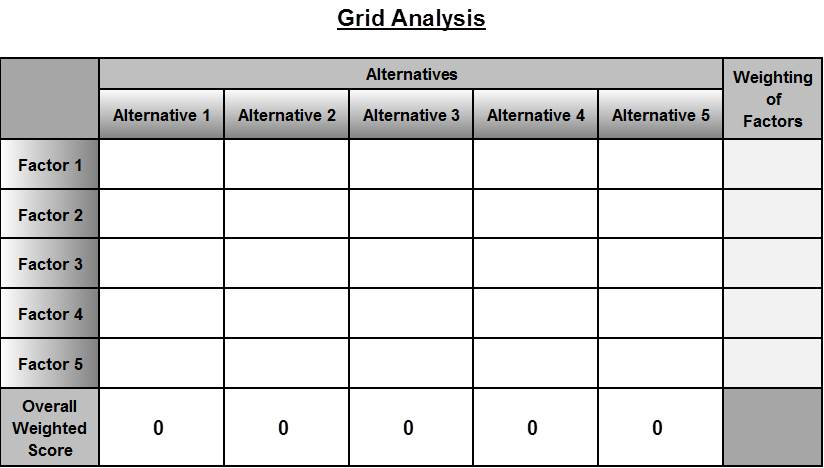Click to go to our Grid Analysis (diagram)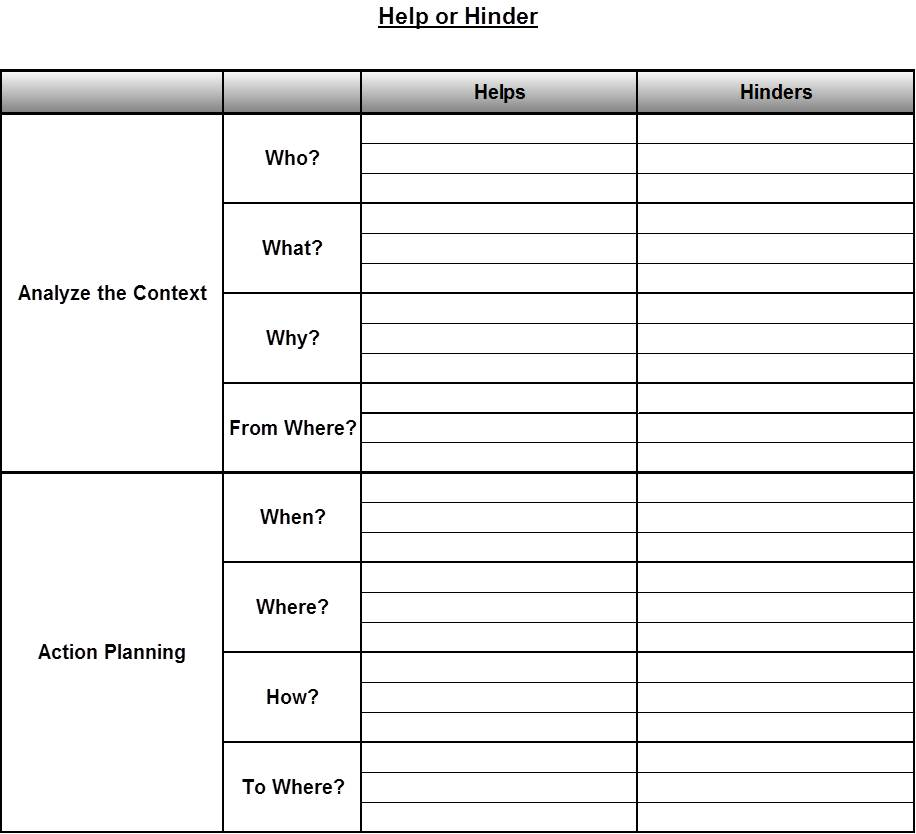Click to go to our Help or Hinder (diagram)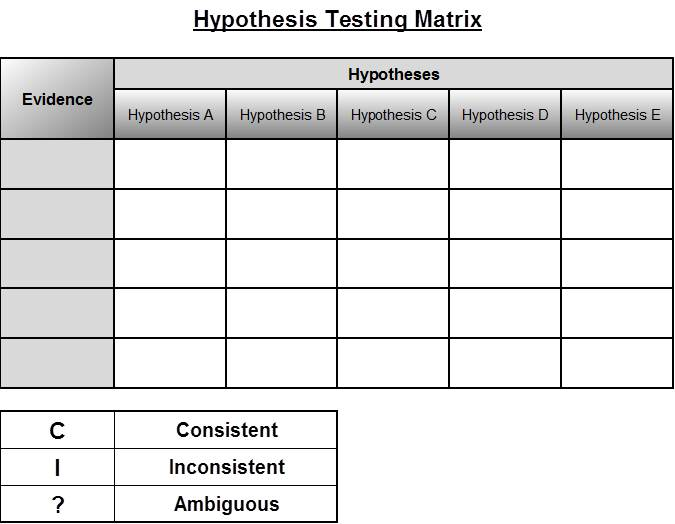Click to go to our Hypothesis Testing Matrix (diagram)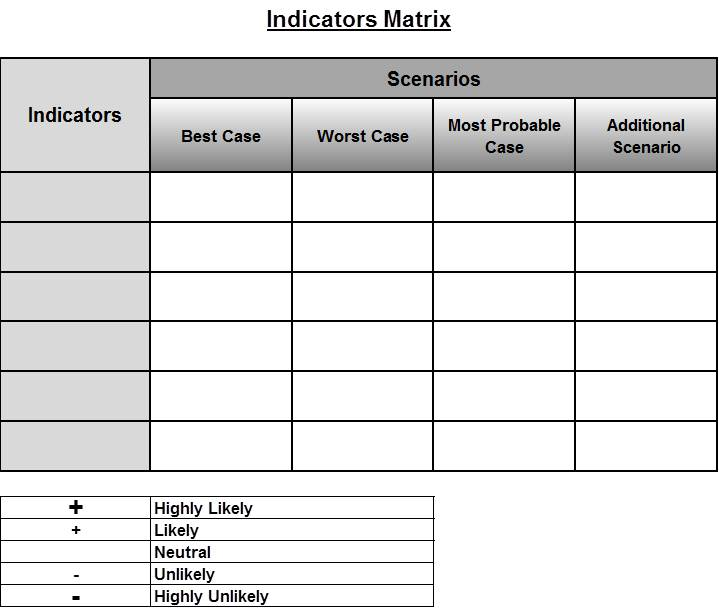Click to go to our Indicators Matrix (diagram)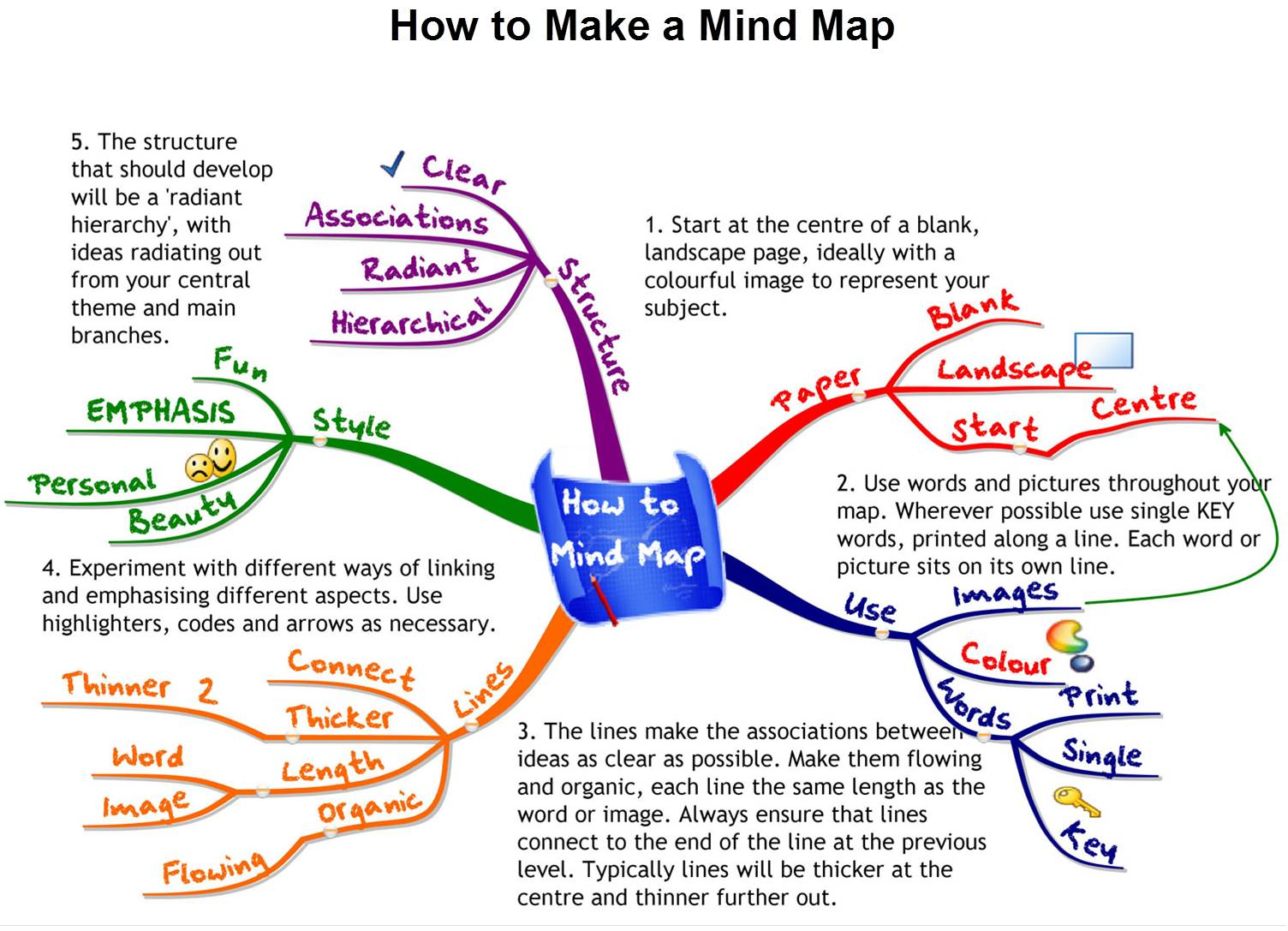Click to go to our Mind Mapping (diagram)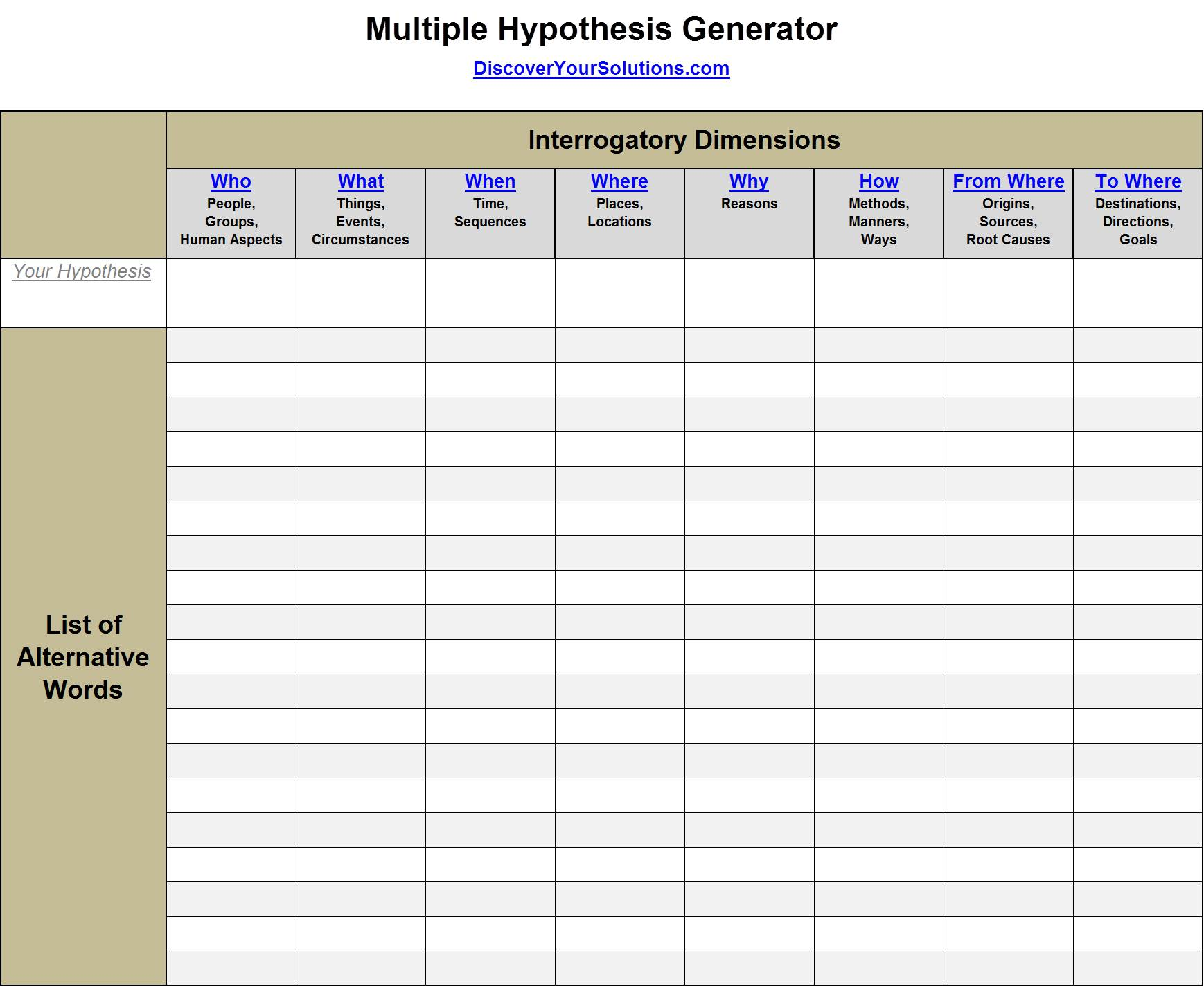Click to go to our Multiple Hypothesis Generator (diagram)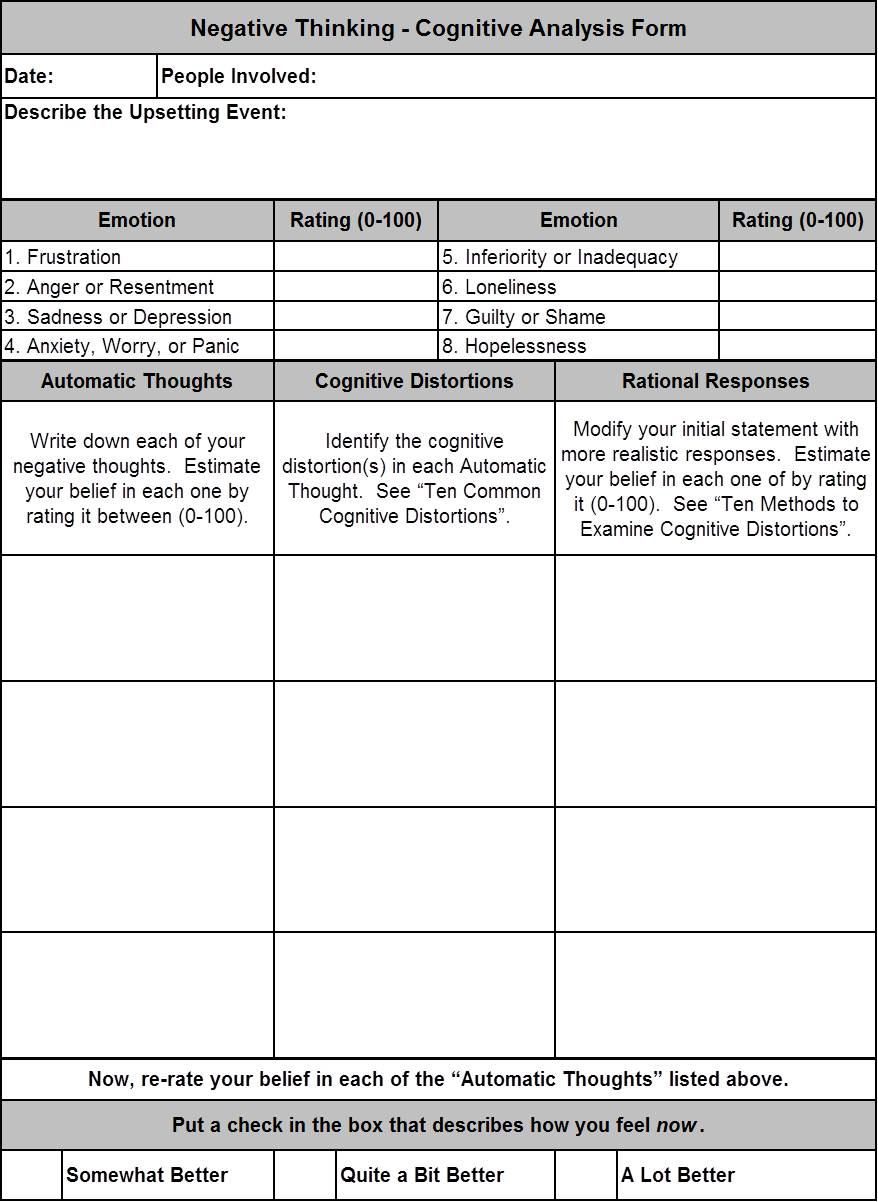Click to go to our Negative Thinking - Cognitive Analysis (diagram)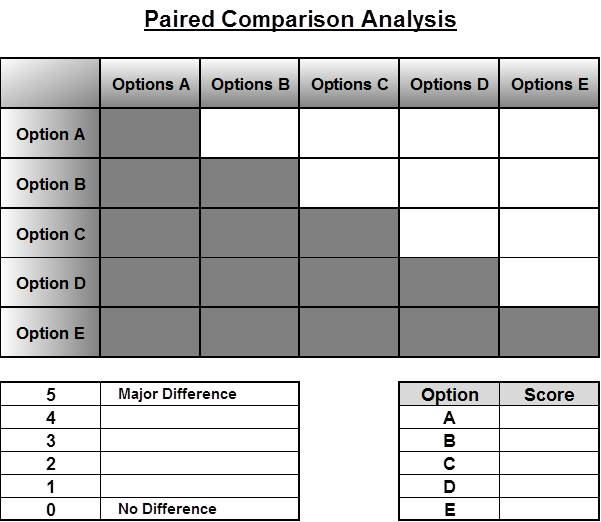Click to go to our Paired Comparison Analysis (diagram)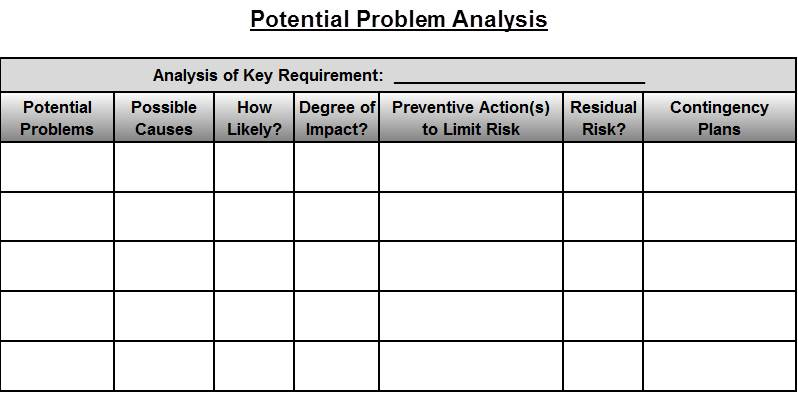Click to go to our Potential Problem Analysis (diagram)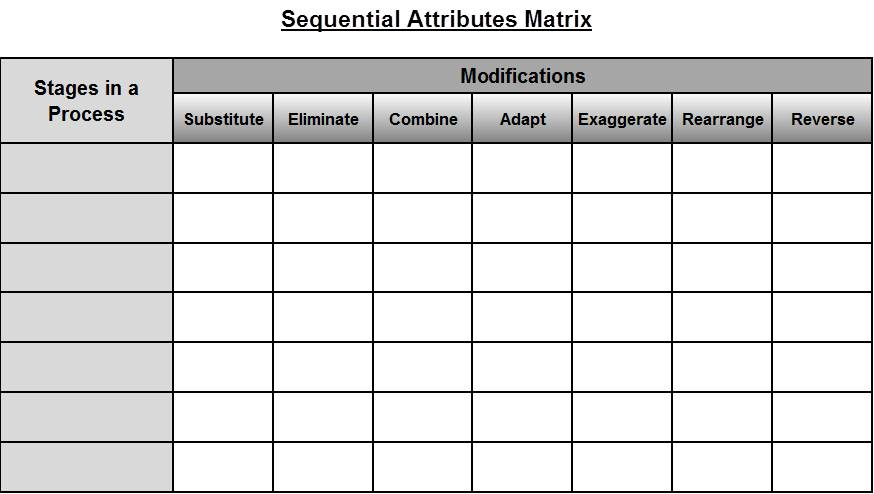Click to go to our Scenarios Matrix (diagram)Click to go to our Sequential Attributes Matrix (diagram)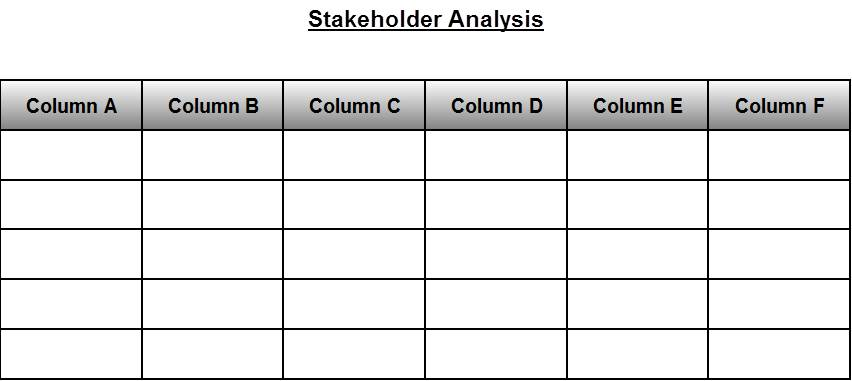Click to go to our Stakeholder Analysis (diagram)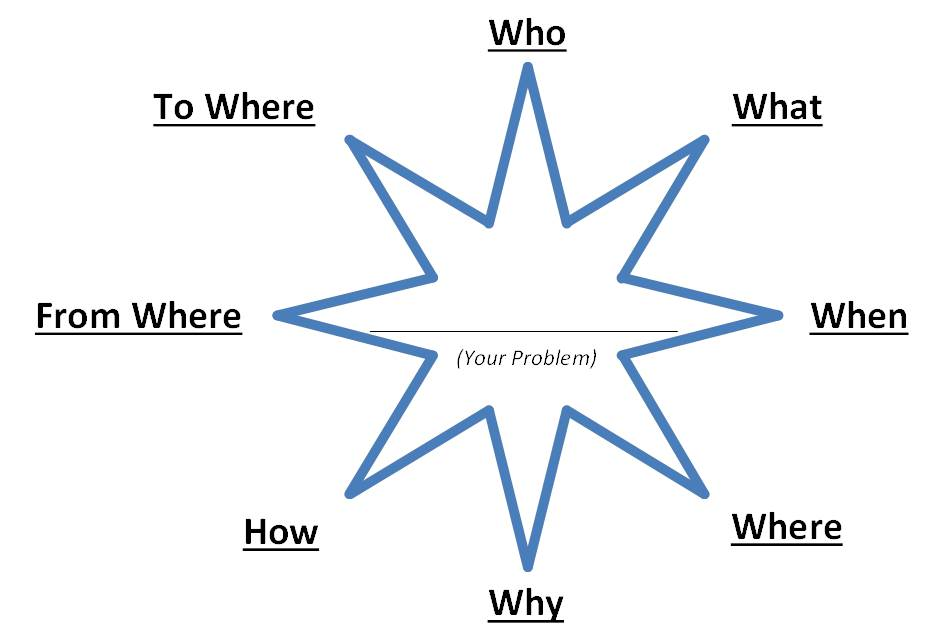Click to go to our Starbursting (diagram)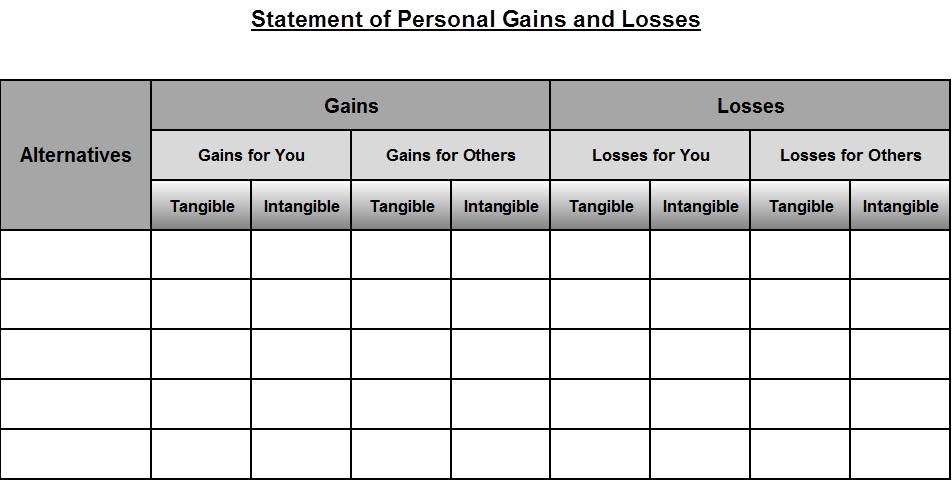Click to go to our Statement of Personal Gains and Losses (diagram)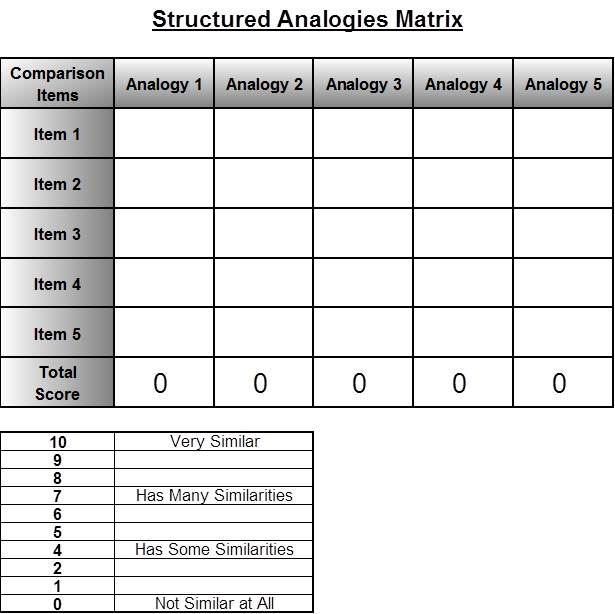Click to go to our Structured Analogies Matrix (diagram)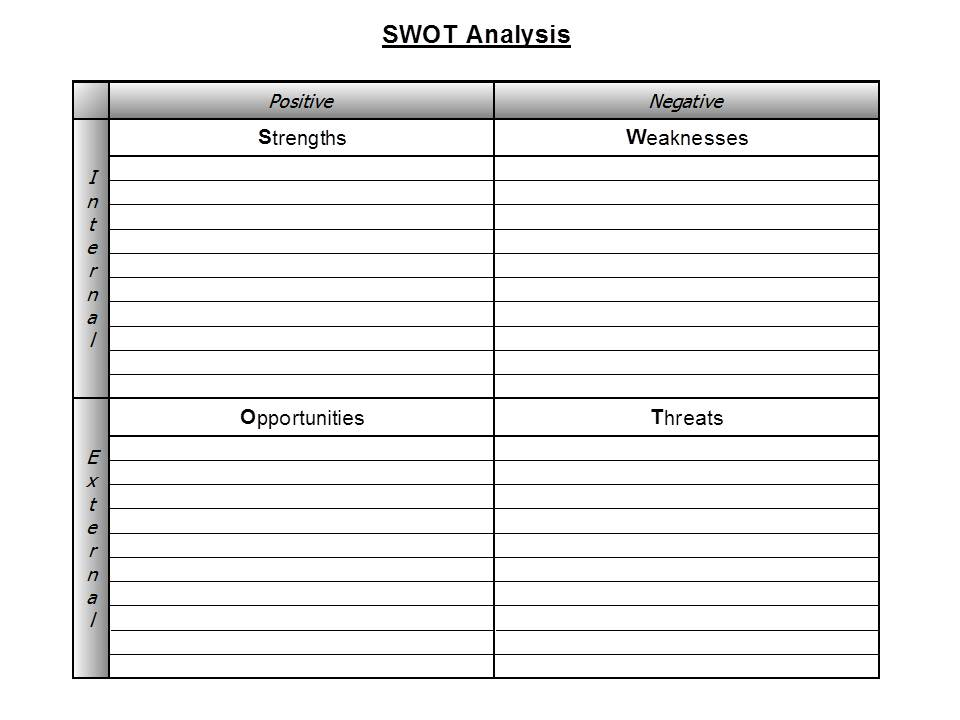Click to go to our SWOT Analysis (diagram)

List Solver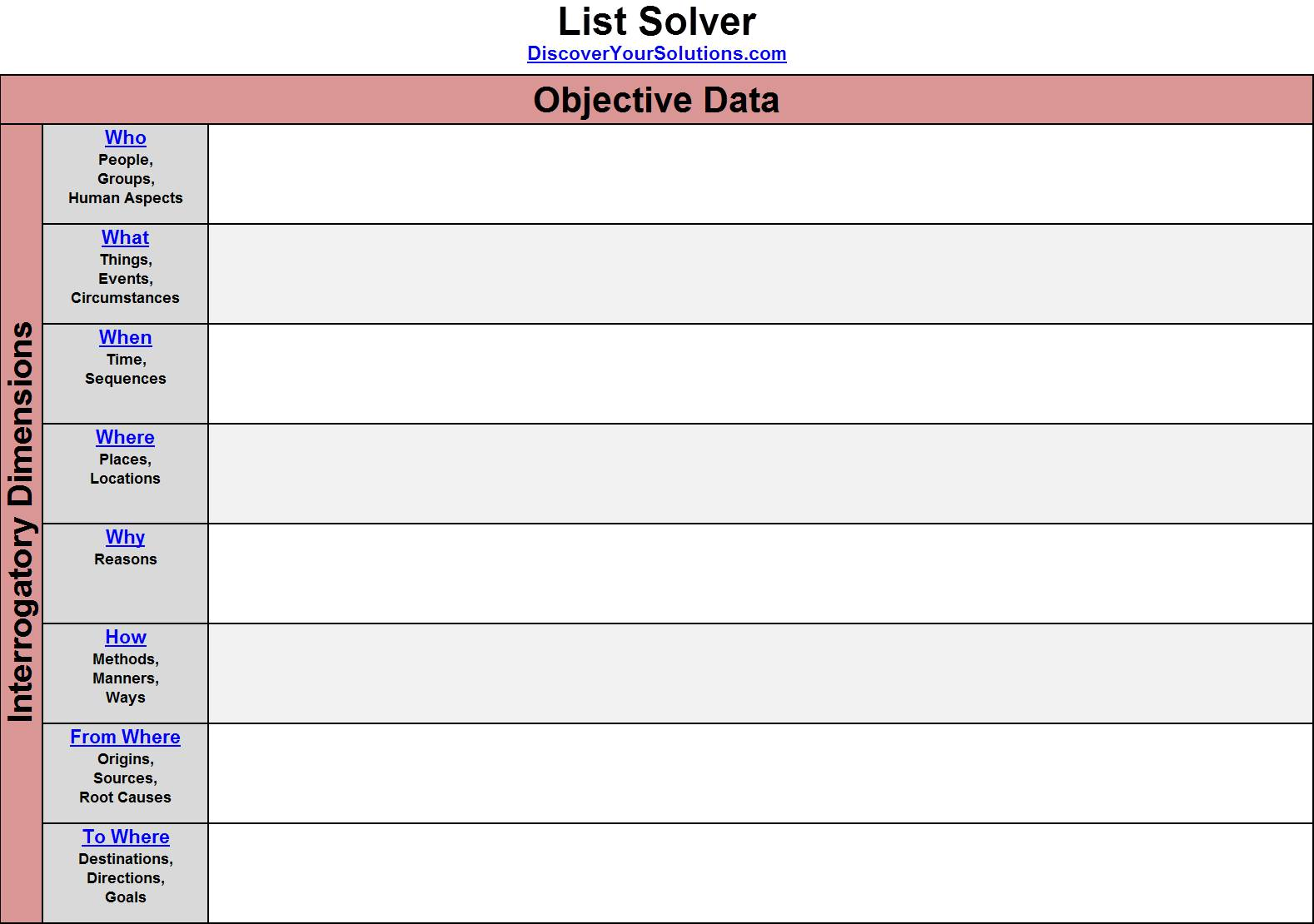Click to go to our List Solver for Objective Data (illustration)Click to go to our List Solver Form for Objective Data (form)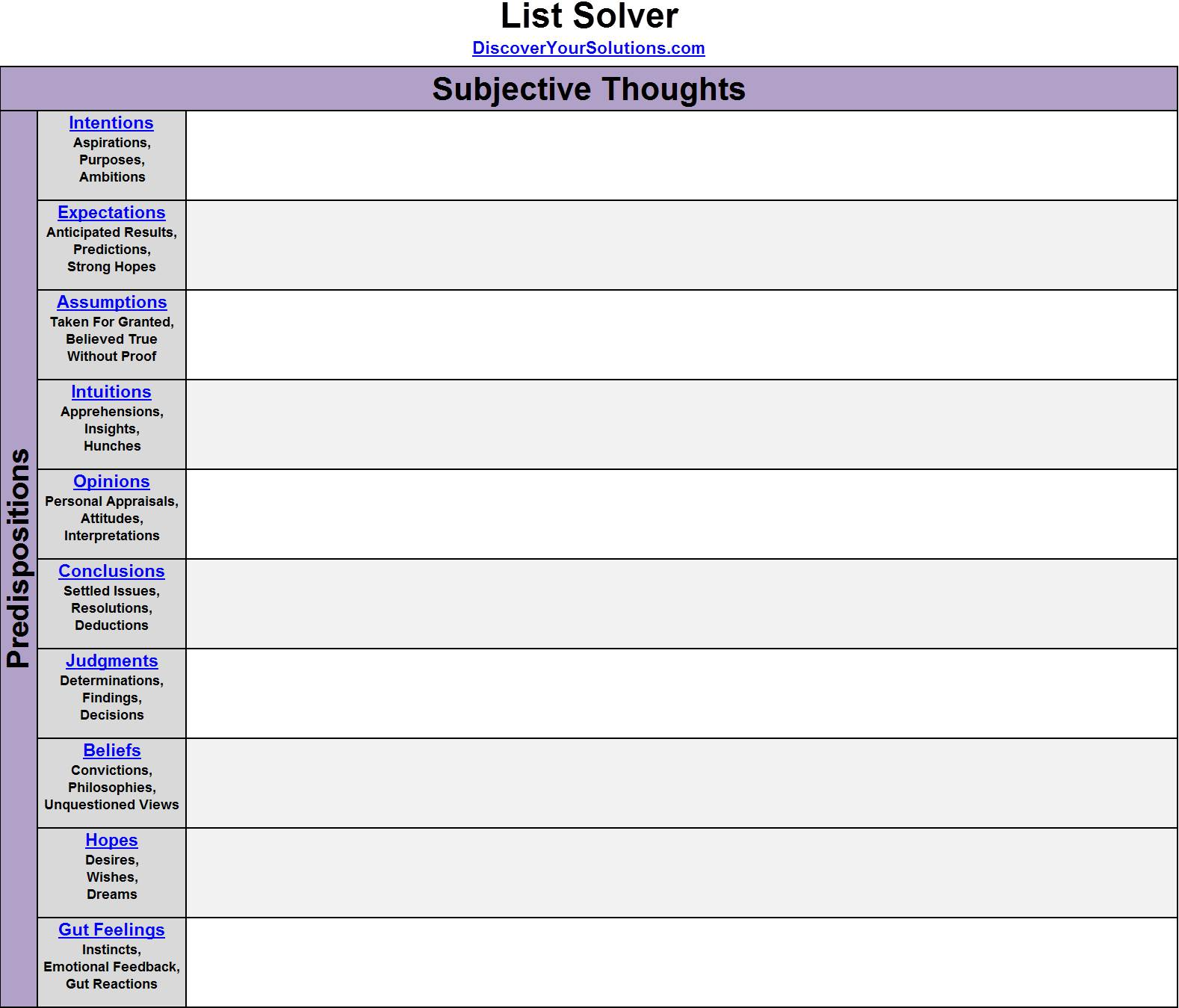Click to go to our List Solver for Subjective Thoughts (illustration)Click to go to our

Problem-Solving Matrix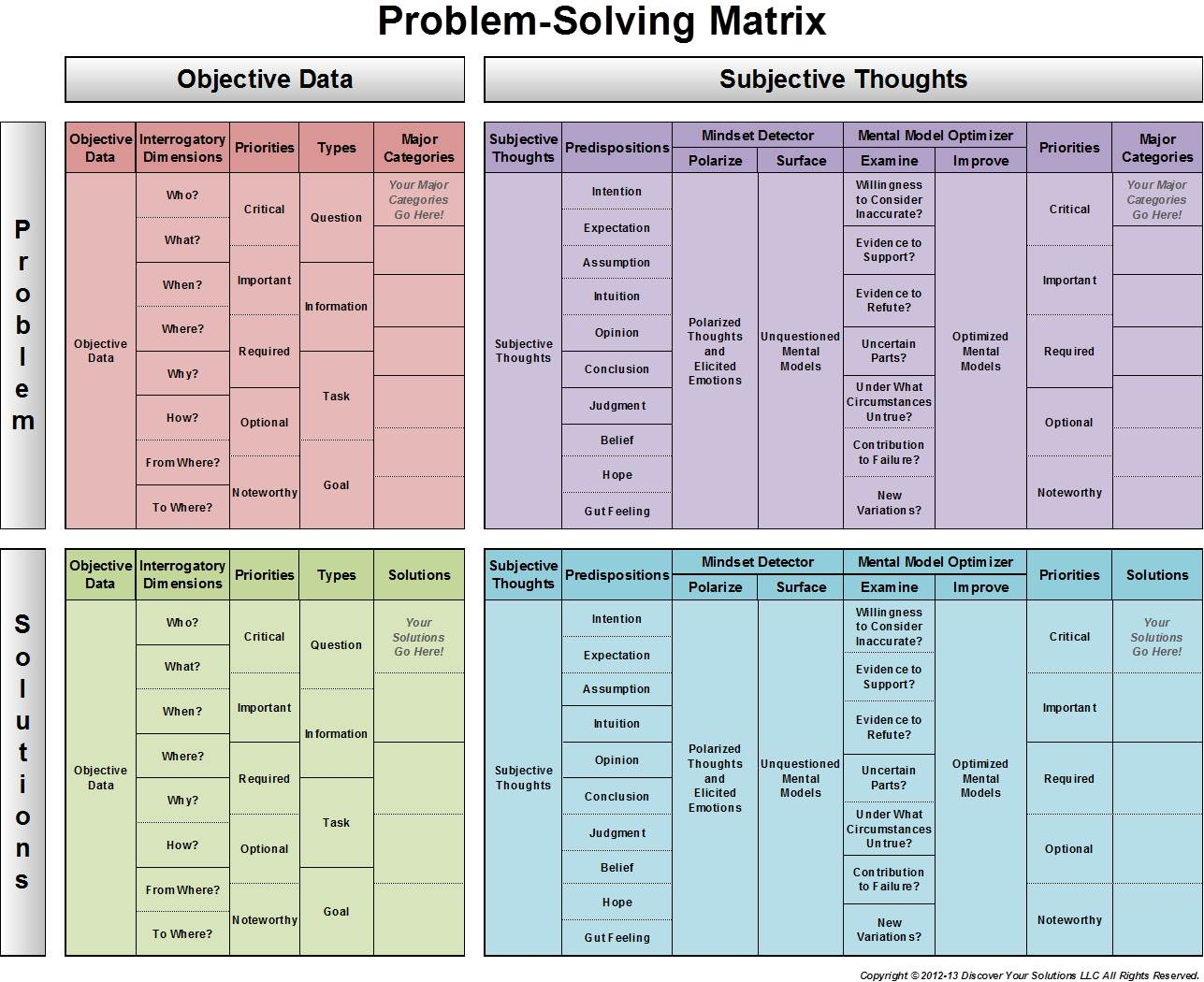Click to go to our Problem-Solving Matrix (illustration)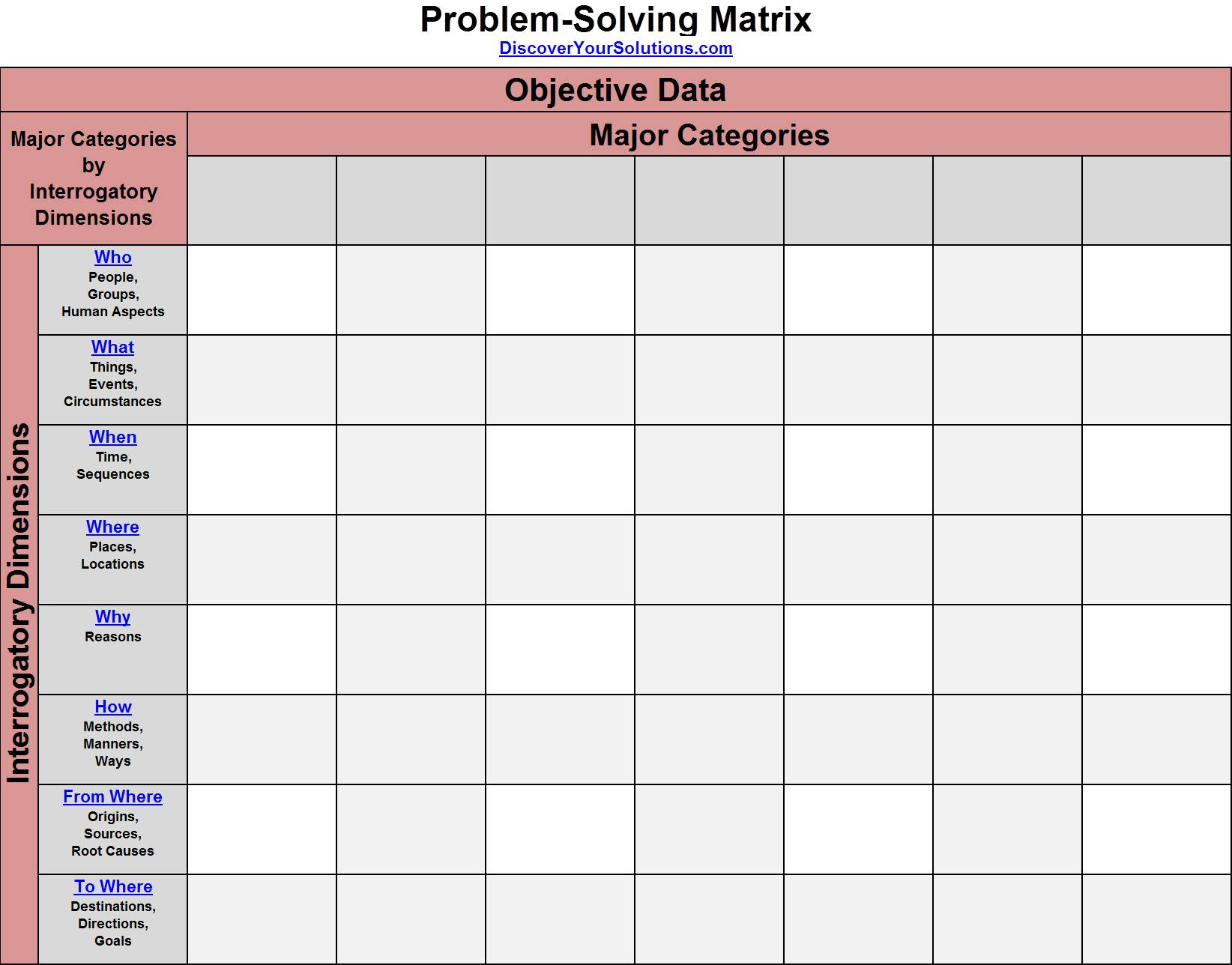Click to go to our Problem-Solving Matrix - Objective Data / Problem (illustration)Click to go to our Matrix Solver Form - Objective Data / Problem (form)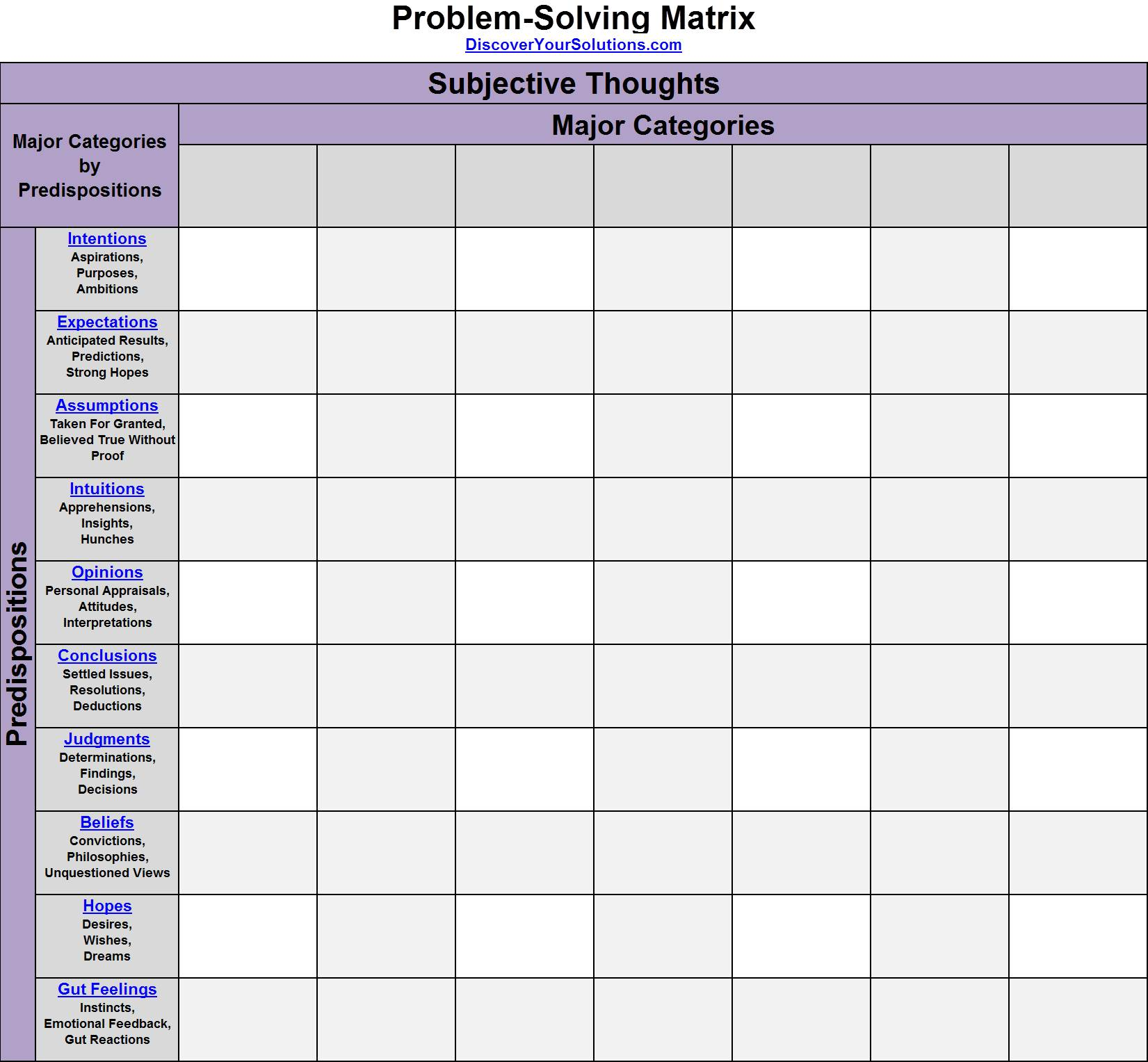Click to go to our Problem-Solving Matrix - Subjective Thoughts / Problem (illustration)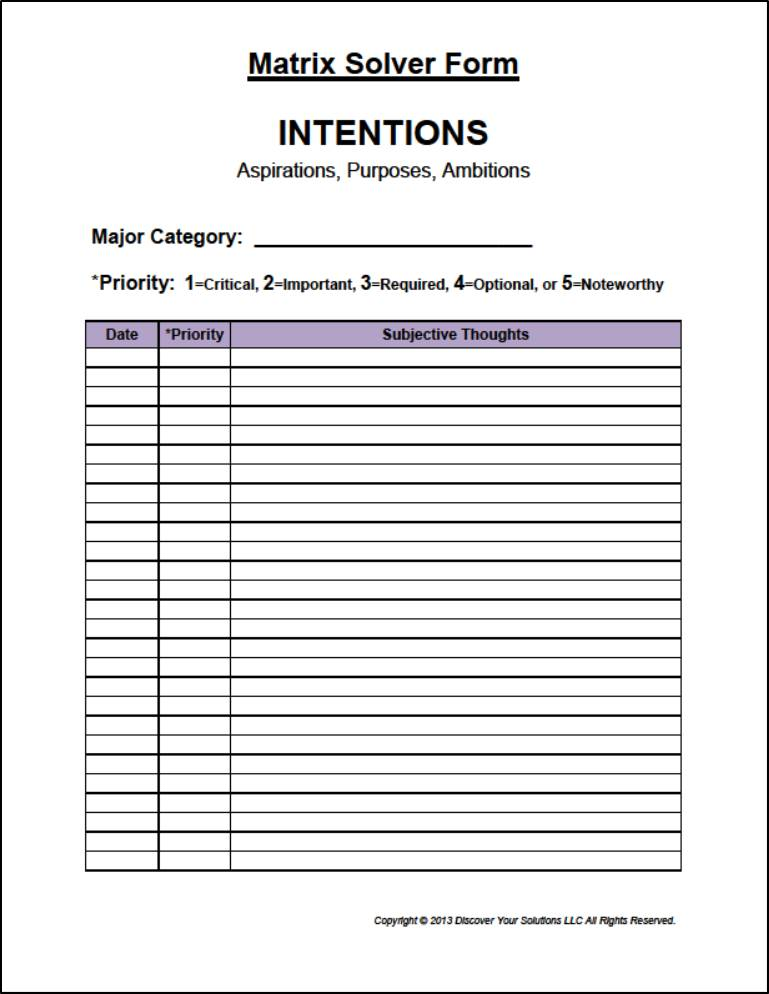Click to go to our Matrix Solver Form - Subjective Thoughts / Problem (form)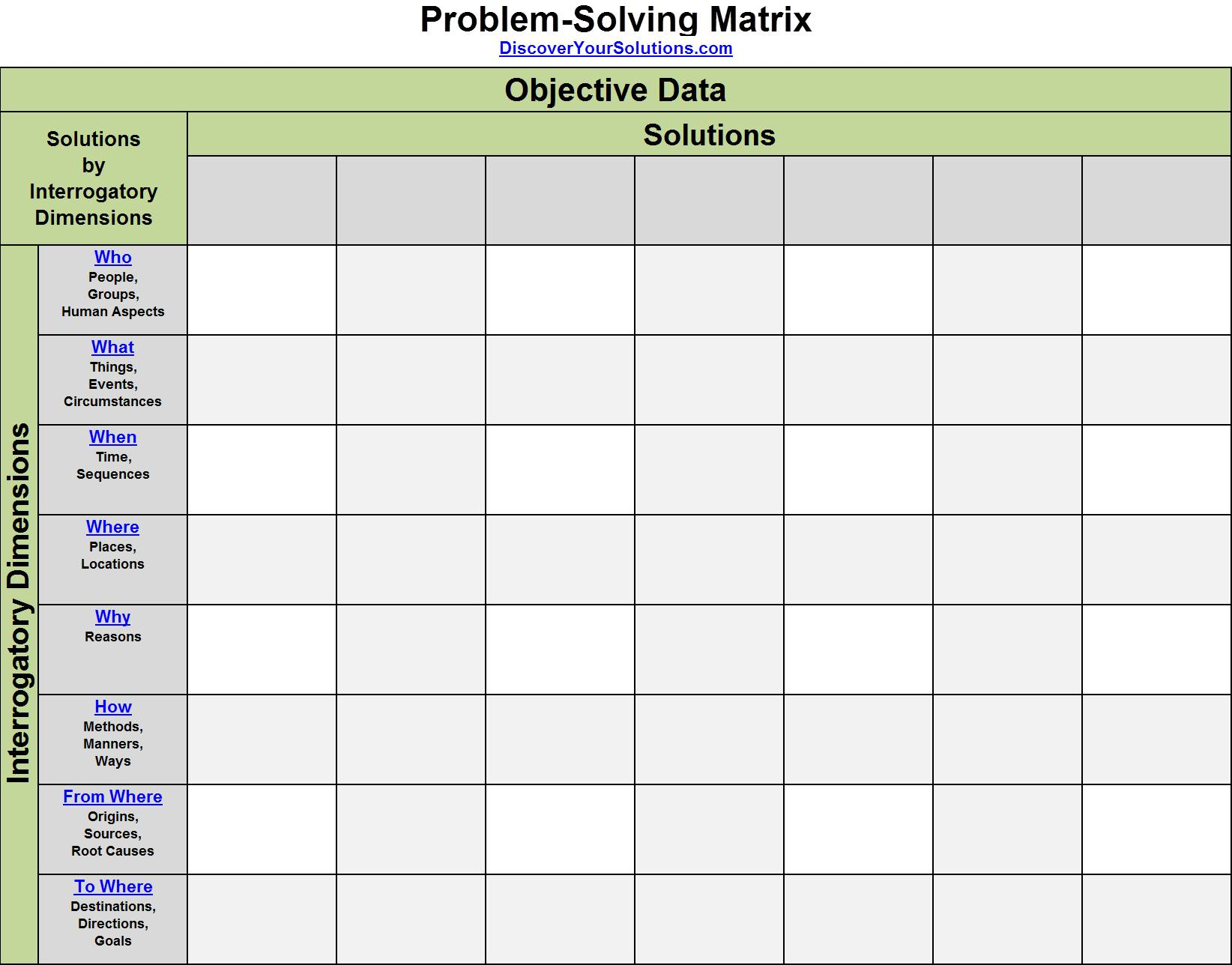Click to go to our Problem-Solving Matrix - Objective Data / Solution (illustration)Click to go to our Matrix Solver Form - Objective Data / Solution (form)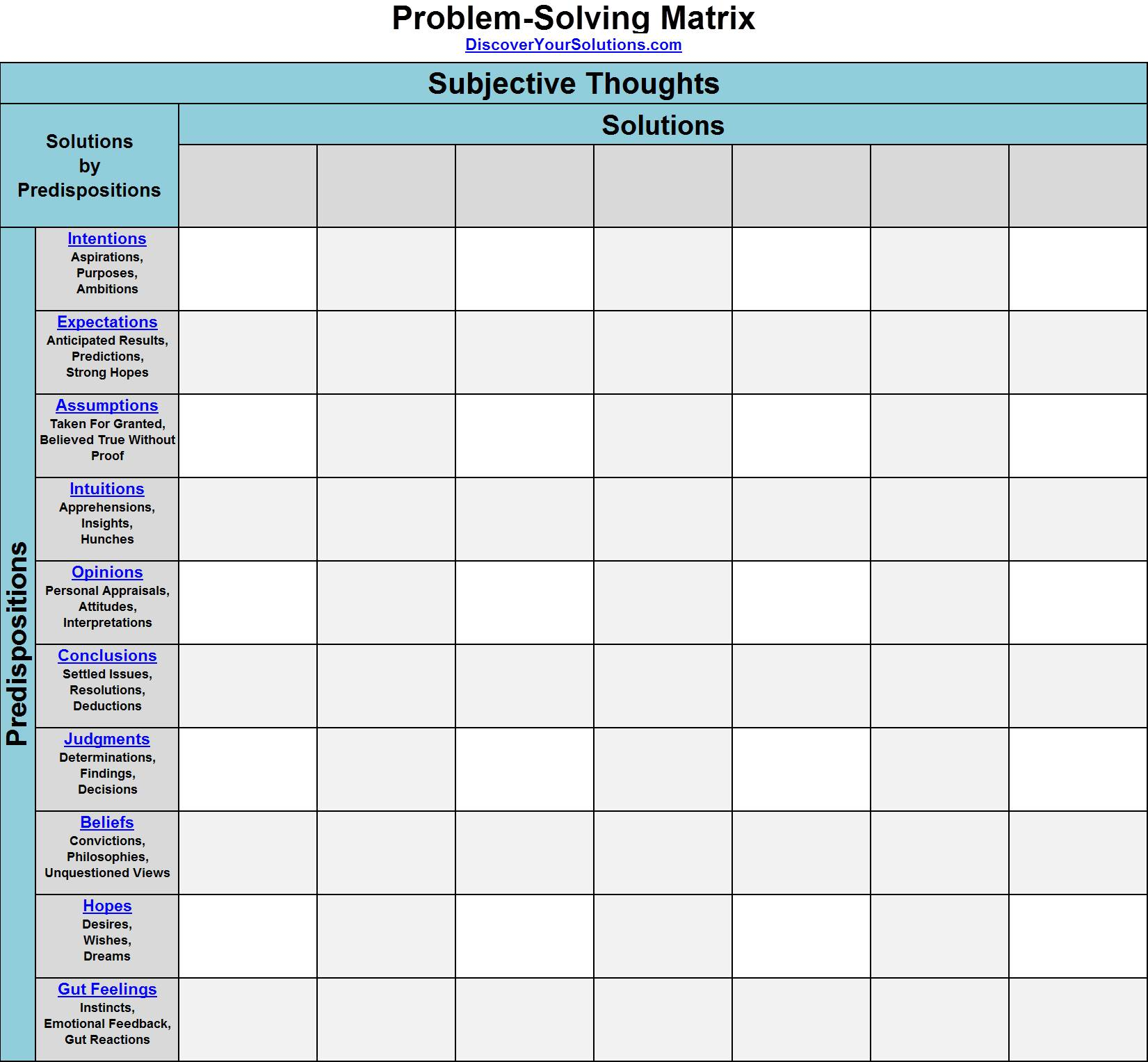Click to go to our Problem-Solving Matrix - Subjective Thoughts / Solution (illustration)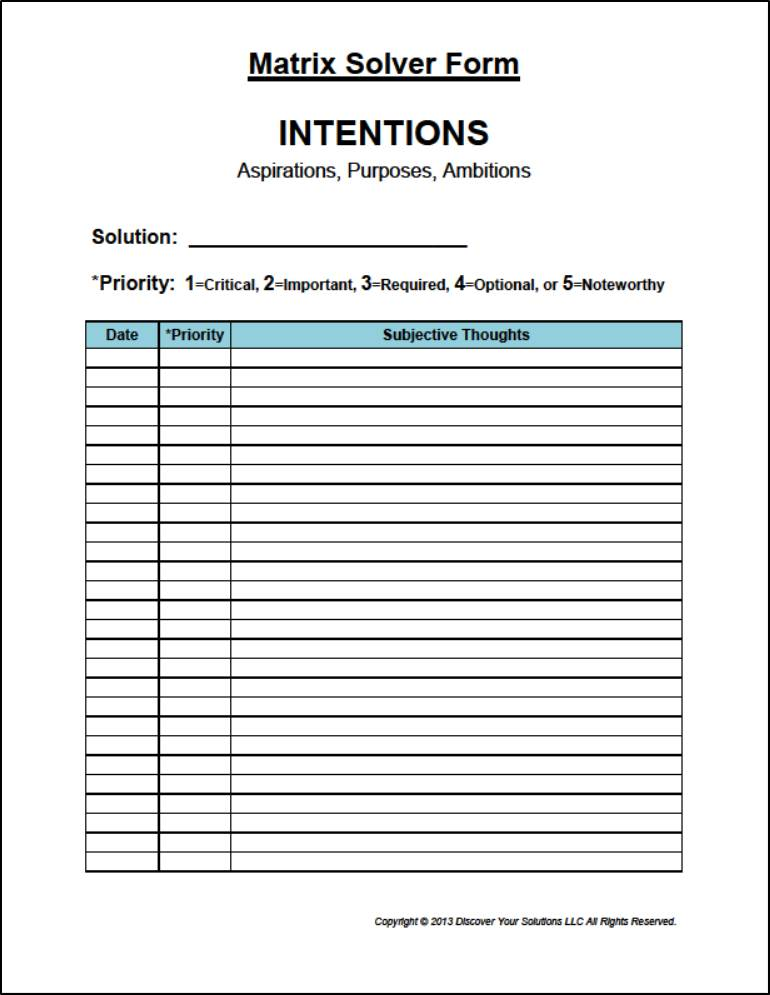Click to go to our Matrix Solver Form - Subjective Thoughts / Solution (form)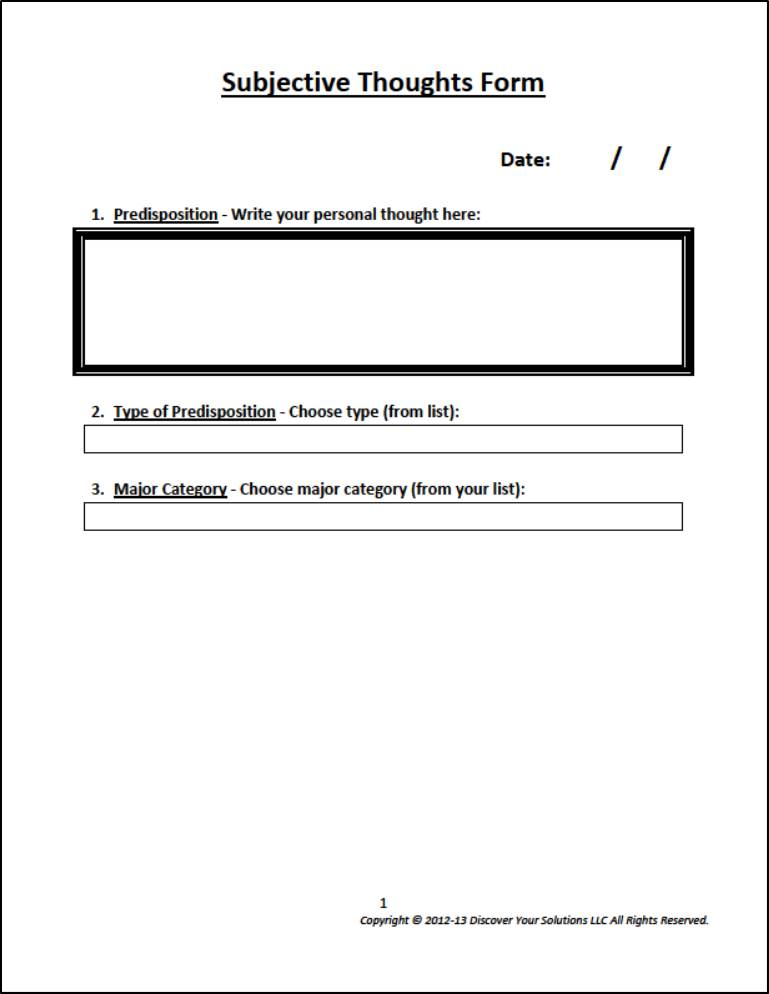Click to go to our Subjective Thoughts Form (form)

Model of Analytic Processes - Objective Data

The 'Model of Analytic Processes - Objective Data' is a conceptual model of how the structure of objective data can be developed over time.Model of Analytic Processes - Subjective Thoughts

The 'Model of Analytic Processes - Subjective Thoughts' is a conceptual model of how the structure of subjective data can be developed over time.Problem Solving Model

Problem solving is a continuous process where you externalize your internal thought processes into a systematic analytical framework. You then internalize the external analytic processes into internal mental models and begin the process again.Solvers Model

Our Solvers are all-in-one integrated methods that combines problem solving tools with cognitive psychology. Our MySolver™ program then leverages this approach with advanced cloud computing. Our systems give you the best opportunity to find your solutions.Bullet Proofing

Diagram of our Bullet Proofing table:Cause and Effect - Fishbone Diagram

Here's an example of a Cause and Effect - Fishbone Diagram for missed free throws:Chronology

Here's a diagram of our Chronology Form:Commitment Chart

Diagram of our Commitment Chart:Cross Impact Matrix

Diagram of our Cross Impact Matrix:Five Whys

Diagram of our Five Whys table:Five Whys Alternative

Diagram of our Five Whys Alternative table:Flow Chart

Diagram of a Flow Chart:Force Field Analysis

Conceptual Diagram of a Force Field Analysis:Grid Analysis

Diagram:  Here's a the diagram our Grid Analysis table.Help or Hinder

Diagram of a Help or Hinder table:Hypothesis Testing Matrix

Diagram:  Here's a diagram of our Hypothesis Testing Matrix.Indicators Matrix

Diagram of our Indicators Matrix:Mind Mapping

Diagram of How to Make a Mind Map:Multiple Hypothesis Generator

Diagram:  Here's a diagram you can use of our Multiple Hypothesis Generator.Negative Thinking - Cognitive Analysis

Diagram of the Negative Thinking - Cognitive Analysis Form:Adapted from The Feeling Good Handbook, by David D. Burns, M.D., New York: Penguin Putnam, 1999.

Paired Comparison Analysis

Diagram:  Here's a the diagram our Paired Comparison Analysis.Potential Problem Analysis

Diagram:  Here's a the diagram our Potential Problem Analysis.Scenarios Matrix

Diagram of a Scenarios Matrix: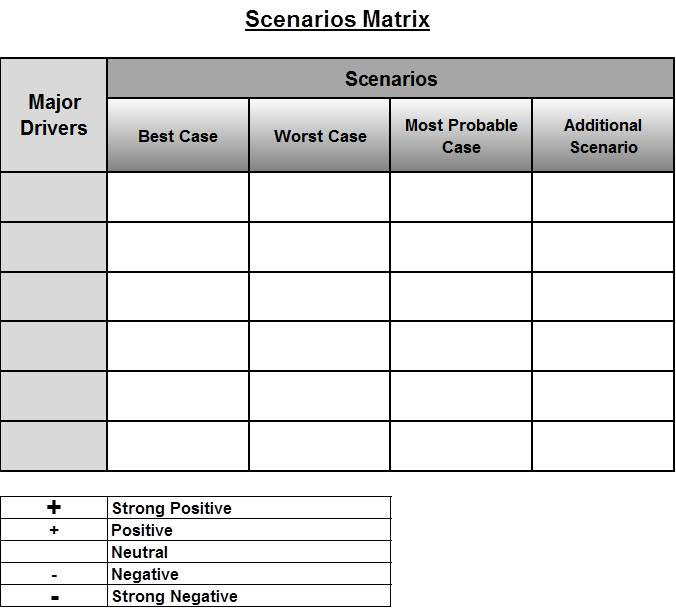Sequential Attributes Matrix

Diagram of a Sequential Attributes Matrix:Stakeholder Analysis

Diagram of a Stakeholder Analysis table:Starbursting

Diagram:  Here's a diagram of our Starbursting tool.Statement of Personal Gains and Losses

Diagram of a Statement of Personal Gains and Losses table:Structured Analogies Matrix

Diagram:  Here's a the diagram our Structured Analogies Matrix.SWOT Analysis

Diagram of our SWOT Analysis:List Solver for Objective Data

Here's a visualization of our List Solver for Objective Data.List Solver Form for Objective DataList Solver for Subjective Thoughts

Here's a visualization of our List Solver for Subjective Thoughts.List Solver Form for Subjective Thoughts

Use our List Solver Form to record the thoughts that you're predisposed to.Problem-Solving Matrix

The Problem-Solving Matrix is the design template used to program the MySolver™. It provides the structural basis for analyzing your problem and your solutions. Many of the theories and models behind the Problem-Solving Matrix are discussed in the Detailed Info section.Problem-Solving Matrix - Objective Data / Problem

Here's a helpful visualization of the 1st Quadrant of the Problem-Solving Matrix - Objective Data / Problem.Matrix Solver Form - Objective Data / ProblemProblem-Solving Matrix - Subjective Thoughts / Problem

Here's a helpful visualization of the 2nd Quadrant of the Problem-Solving Matrix - Subjective Thoughts / Problem.Matrix Solver Form - Subjective Thoughts / Problem

Use our Matrix Solver Form to record your Predispositions for each Major Category of your Problem.Problem-Solving Matrix - Objective Data / Solutions

Here's a helpful visualization of the 3rd Quadrant of the Problem-Solving Matrix - Objective Data / Solutions.Matrix Solver Form - Objective Data / SolutionsProblem-Solving Matrix - Subjective Thoughts / Solutions

Here's a helpful visualization of the 4th Quadrant of the Problem-Solving Matrix - Subjective Thoughts / Solutions.Matrix Solver Form - Subjective Thoughts / Solutions

Use our Matrix Solver Form to record your Predispositions for each one of your Solutions.